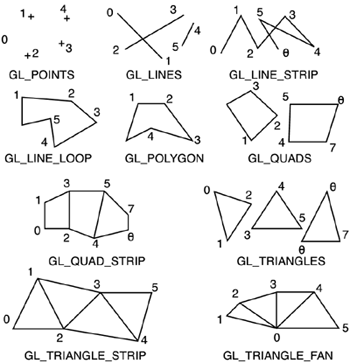# Immediate Mode Rendering

OpenGL decomposes any complex object into a series of simple primitives: points, lines, triangles, and so on. The reason for this is that graphics hardware is very good at rendering simple objects. Hardware routines for rendering triangles or lines are thousands of times faster than software implementations. More complex objects are unsupported because of the large variety (and hence algorithmic complexity).

Objects can be declared to OpenGL using a variety of rendering methods. Because we are just starting with OpenGL, I will cover only the simplest (and sadly, least efficient) rendering method, leaving more involved constructs for subsequent sections. The method we will be using is called immediate mode rendering, and involves sending the data to the hardware using many calls, one per element. Immediate mode rendering blocks are always started with the glBegin call, which specifies what kind of primitive we want to render, and should finish with a call to glEnd. The calls between Begin and End specify the geometric data. Remember that you can batch as many primitives as you want in a Begin-End construct: the more primitives, the better the performance.

As a summary, here is a list of the geometric primitives available in OpenGL, along with how data is interpreted in each one. You can see a graphic representation of each primitive in Figure B.2.

• GL_POINTS Draws a point at each of the n vertices.

• GL_LINES Draws a series of unconnected line segments. Segments are drawn between v0 and v1, between v2 and v3, and so on. If n is odd, the last segment is drawn between vn-3 and vn-2, and vn-1 is ignored.

• GL_POLYGON Draws a polygon using the points v0, …, vn-1 as vertices. n must be at least 3, or nothing is drawn. In addition, the polygon specified must not intersect itself and must be convex. If the vertices don't satisfy these conditions, the results are unpredictable.

• GL_TRIANGLES Draws a series of triangles (three-sided polygons) using vertices v0, v1, v2, then v3, v4, v5, and so on. If n isn't an exact multiple of 3, the final one or two vertices are ignored.

• GL_LINE_STRIP Draws a line segment from v0 to v1, then from v1 to v2, and so on, finally drawing the segment from vn-2 to vn-1. Thus, a total of n-1 line segments are drawn. Nothing is drawn unless n is larger than 1. There are no restrictions on the vertices describing a line strip (or a line loop); the lines can intersect arbitrarily.

• GL_LINE_LOOP Same as GL_LINE_STRIP except that a final line segment is drawn from vn-1 to v0, completing a loop.

• GL_QUADS Draws a series of quadrilaterals (four-sided polygons) using vertices v0, v1, v2, v3, then v4, v5, v6, v7, and so on. If n isn't a multiple of 4, the final one, two, or three vertices are ignored.

• GL_QUAD_STRIP Draws a series of quadrilaterals (four-sided polygons) beginning with v0, v1, v3, v2, then v2, v3, v5, v4, then v4, v5, v7, v6, and so on. See Figure B.2. n must be at least 4 before anything is drawn, and if n is odd, the final vertex is ignored.

• GL_TRIANGLE_STRIP Draws a series of triangles (three-sided polygons) using vertices v0, v1, v2, then v2, v1, v3 (note the order), then v2, v3, v4, and so on. The ordering is to ensure that the triangles are all drawn with the same orientation so that the strip can correctly form part of a surface. Figure B.2 should make the reason for the ordering obvious. n must be at least 3 for anything to be drawn.

• GL_TRIANGLE_FAN Same as GL_TRIANGLE_STRIP except that the vertices are v0, v1, v2, then v0, v2, v3, then v0, v3, v4, and so on. See Figure B.2.

##### Figure B.2. OpenGL's primitive types.Each vertex in a rendering batch can be assigned a color, texture coordinates, normal, and so on. To begin with, colors are specified with the glColor* family of calls. You must keep in mind that OpenGL colors are active after the respective glColor call, so you need to specify the color prior to sending the vertex to the hardware. Additionally, colors are persistent: All vertices in a batch will use the last declared color until a new one is assigned. For example, the following code paints a triangle:

` glBegin(GL_TRIANGLES); glColor3f(1,0,0); glVertex3f(-1,0,0); glColor3f(1,1,0); glVertex3f(1,0,0); glVertex3f(0,1,0); glEnd(); `

The first vertex will be red (1,0,0). The second and third will be yellow. As an efficiency concern when using immediate mode rendering, remember to batch primitives together when you can. The following two code snippets, although similar, yield very different performance:

` for (i=0;i<1000;i++)    {    glBegin(GL_QUADS);    // paint a quad here    glEnd();    } glBegin(GL_QUADS); for (i=0;i<1000;i++)    {    // paint a quad here    } glEnd(); `

In the first case, we are painting 1,000 primitives and sending 2,000 OpenGL calls (each one with its overhead) to the pipeline, and that's not counting the rendering itself. In the second code snippet, we are sending one large batch of primitives and doing only two OpenGL calls. The second code snippet is significantly faster than the first. As you will soon see, there are very few reasons to break a primitive batch, and those reasons are usually performing a transform or changing the texture map. Other than that, we should create batches as big as possible.Core Techniques and Algorithms in Game Programming2003
ISBN: N/A
EAN: N/A
Year: 2004
Pages: 261